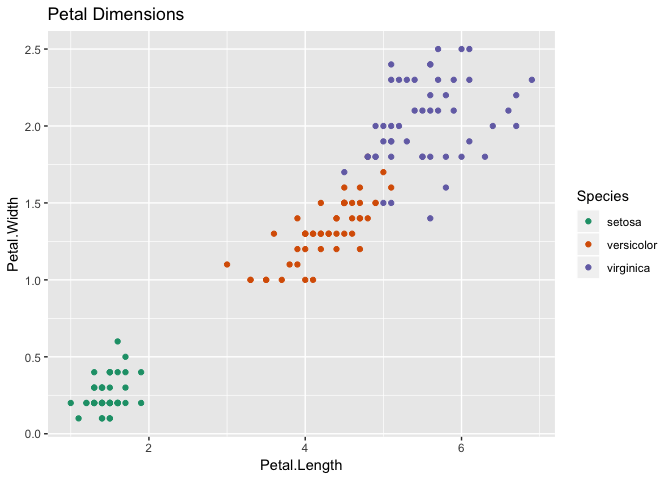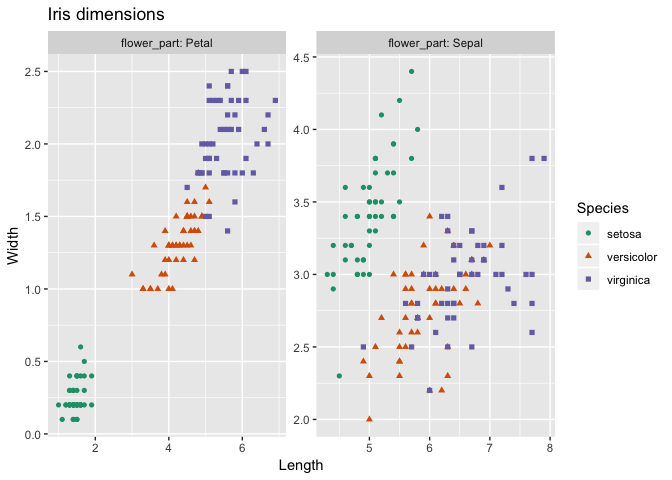# Faceted Graphs with cdata and ggplot2

In between client work, John and I have been busy working on our book, Practical Data Science with R, 2nd Edition. To demonstrate a toy example for the section I’m working on, I needed scatter plots of the petal and sepal dimensions of the `iris` data, like so:I wanted a plot for petal dimensions and sepal dimensions, but I also felt that two plots took up too much space. So, I thought, why not make a faceted graph that shows both:Except — which columns do I plot and what do I facet on?

``head(iris)``
``````##   Sepal.Length Sepal.Width Petal.Length Petal.Width Species
## 1          5.1         3.5          1.4         0.2  setosa
## 2          4.9         3.0          1.4         0.2  setosa
## 3          4.7         3.2          1.3         0.2  setosa
## 4          4.6         3.1          1.5         0.2  setosa
## 5          5.0         3.6          1.4         0.2  setosa
## 6          5.4         3.9          1.7         0.4  setosa``````

Here’s one way to create the plot I want, using the `cdata` package along with `ggplot2`.

First, load the packages and data:

``````library("ggplot2")
library("cdata")

iris <- data.frame(iris)``````

Now define the data-shaping transform, or control table. The control table is basically a picture that sketches out the final data shape that I want. I want to specify the `x` and `y` columns of the plot (call these the value columns of the data frame) and the column that I am faceting by (call this the key column of the data frame). And I also need to specify how the key and value columns relate to the existing columns of the original data frame.

Here’s what the control table looks like:The control table specifies that the new data frame will have the columns `flower_part`, `Length` and `Width`. Every row of `iris` will produce two rows in the new data frame: one with a `flower_part` value of `Petal`, and another with a `flower_part` value of `Sepal`. The `Petal` row will take the `Petal.Length` and `Petal.Width` values in the `Length` and `Width` columns respectively. Similarly for the `Sepal` row.

Here I create the control table in R, using the convenience function `wrapr::build_frame()` to create the `controlTable` data frame in a legible way.

``````(controlTable <- wrapr::build_frame(
"flower_part", "Length"      , "Width"       |
"Petal"      , "Petal.Length", "Petal.Width" |
"Sepal"      , "Sepal.Length", "Sepal.Width" ))``````
``````##   flower_part       Length       Width
## 1       Petal Petal.Length Petal.Width
## 2       Sepal Sepal.Length Sepal.Width``````

Now I apply the transform to `iris` using the function `rowrecs_to_blocks()`. I also want to carry along the `Species` column so I can color the scatterplot points by species.

``````iris_aug <- rowrecs_to_blocks(
iris,
controlTable,
columnsToCopy = c("Species"))

``````##   Species flower_part Length Width
## 1  setosa       Petal    1.4   0.2
## 2  setosa       Sepal    5.1   3.5
## 3  setosa       Petal    1.4   0.2
## 4  setosa       Sepal    4.9   3.0
## 5  setosa       Petal    1.3   0.2
## 6  setosa       Sepal    4.7   3.2``````

And now I can create the plot!

``````ggplot(iris_aug, aes(x=Length, y=Width)) +
geom_point(aes(color=Species, shape=Species)) +
facet_wrap(~flower_part, labeller = label_both, scale = "free") +
ggtitle("Iris dimensions") +
scale_color_brewer(palette = "Dark2")``````In the next post in this series, I will show how to use `cdata` and `ggplot2` to create a scatterplot matrix.

Categories: Programming Tutorials

Tagged as:### Nina Zumel

Data scientist with Win Vector LLC. I also dance, read ghost stories and folklore, and sometimes blog about it all.

### 8 replies ›

1.Justace Clutter says:

The following is an alternative method using only the tidy verse packages:

```library(tidyverse)

d = iris %>%
mutate(row = seq(1, n())) %>%
group_by(row) %>%
nest() %>%
mutate(keep = map(data, function(x) {
x %>%
gather('type', 'value', contains('.')) %>%
separate('type', c('flower_part', 'measure'), sep = '[.]') %>%
})) %>%
dplyr::select(-row, -data) %>%
unnest(keep)

ggplot(d, aes(x=Length, y=Width)) +
geom_point(aes(color=Species, shape=Species)) +
facet_wrap(~flower_part, labeller = label_both, scale = "free") +
ggtitle("Iris dimensions") +
scale_color_brewer(palette = "Dark2")
```
2.Barry Rowlingson says:

This example can be done with one `rbind` and creating one trivial new column. I can also see the value in having a programmatic way of doing it using `cdata` to write a specification. But that mess of percentage sandwiches masquerading as “tidy”? It has pipes within functions within pipes. Where’s the value in that?

1.John Mount says:

The `cdata` system actually gives one extra additional ability to reason about these transforms.

For example: for this direction (`rowrecs_to_blocks()`) any two row control table is an `rbind` in disguise (as this is), and any two column control table is a `tidyr::gather()` (so this isn’t a single `tidyr::gather()`).

In fact the control table is literally the column re-namings you would do to solve this with an an rbind; but written in a very orderly and concise format, check out how the colored arguments match in the diagram comparing two solutions, below.

Also in terms of Codd-style data manipulation algebra: `rowrecs_to_blocks()` is analogous to joining with the control table, and `blocks_to_rowrecs()` is its inverse (and looks a lot like a table widening followed by a summary/coalesce).

Some articles and video developing the theory:

3.Mark van der Loo (@markvdloo) says:

There is indeed a tidyverse solution, but it is easier than what Justin proposes:

```library(dplyr)
library(tidyr)
library(ggplot2)

out <- iris %>%
gather(flower_part, Value, -Species,-Key) %>%      # numeric data in long form
separate(flower_part,into=c("Part","Measure")) %>% # Separate column with Length/width
spread(Measure, Value)                             # put Length/Width in columns

out %>% ggplot(aes(x=Width, y=Length,col=Species, shape=Species)) +
facet_grid(~Part) + geom_point()
```
1.Justace Clutter says:

Ahh. I felt there was a simpler method I just was not coming up with it. Thanks for the example.

4.John Mount says:

We now have an article up detailing more on the systematic method to derive the transform control table for a given desired transform here.

5.Paul says:

Thanks for the post. Also could be accomplished with 3 sqldf statements

septal = sqldf(‘select Species, “Septal” as flower_part, “Sepal.Length” as length, “Sepal.Width” as width from iris ‘)

petal = sqldf(‘select Species, “Petal” as flower_part, “Petal.Length” as length, “Petal.Width” as width from iris ‘)

iris_aug = sqldf(“select * from septal
union all
select * from petal”)

But good to have other tools available and I have not used cdata much. The concept of a control table is nice.

1.John Mount says:

In fact `cdata` itself can translate the control table into `SQL` code realizing the transform (and thus be used with databases).

```library("cdata")
library("rquery")

controlTable <- wrapr::build_frame(
"flower_part", "Length"      , "Width"       |
"Petal"      , "Petal.Length", "Petal.Width" |
"Sepal"      , "Sepal.Length", "Sepal.Width" )

columnsToCopy = "Species"

db <- DBI::dbConnect(RSQLite::SQLite(),
":memory:")

iris_r <- rquery::rq_copy_to(db, "iris", iris)

res_tab <- rowrecs_to_blocks_q(
iris_r\$table_name,
controlTable = controlTable,
my_db = db,
columnsToCopy = columnsToCopy,
showQuery = TRUE)

#  "CREATE  TABLE `mvtrq_03508568885469357450_0000000001` AS  SELECT a.`Species`, b.`flower_part`, CASE  WHEN b.`flower_part` = 'Petal' THEN a.`Petal.Length`  WHEN b.`flower_part` = 'Sepal' THEN a.`Sepal.Length` ELSE NULL END AS `Length`, CASE  WHEN b.`flower_part` = 'Petal' THEN a.`Petal.Width`  WHEN b.`flower_part` = 'Sepal' THEN a.`Sepal.Width` ELSE NULL END AS `Width` FROM `iris` a CROSS JOIN `mvtrq_03508568885469357450_0000000000` b "

rquery::rstr(db, res_tab)

# table `mvtrq_03508568885469357450_0000000001` SQLiteConnection
#  nrow: 300
#  NOTE: "obs" below is count of sample, not number of rows of data.
# 'data.frame':   10 obs. of  4 variables:
#  \$ Species    : chr  "setosa" "setosa" "setosa" "setosa" ...
#  \$ flower_part: chr  "Petal" "Sepal" "Petal" "Sepal" ...
#  \$ Length     : num  1.4 5.1 1.4 4.9 1.3 4.7 1.5 4.6 1.4 5
# \$ Width      : num  0.2 3.5 0.2 3 0.2 3.2 0.2 3.1 0.2 3.6

# # or execute as a an rquery pipeline
# iris_r %.>%
#   rowrecs_to_blocks(
#     .,
#     controlTable = controlTable,
#     columnsToCopy = columnsToCopy) %.>%
#   execute(db, .)
```